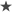Рейтинг:  0 / 5Here is how the US Environmental Protection Agency calculates it:

For PM2.5 between 0 and 12, the AQI is linear between 0 and 50

For PM2.5 between 12.1 and 35.4, the AQI is linear between 51 and 100

For PM2.5 between 35.5 and 55.4, the AQI is linear between 101 and 150

For PM2.5 between 55.5 and 150.4, the AQI is linear between 151 and 200

For PM2.5 between 150.5 and 250.4, the AQI is linear between 201 and 300

For PM2.5 between 250.5 and 350.4, the AQI is linear between 301 and 400

For PM2.5 between 350.5 and 500.4, the AQI is linear between 401 and 500

For PM2.5 greater than 500, AQI is not officially defined, but since such levels have been occurring throughout China in recent years, one of two conventions is used.  Either the AQI is defined as equal to PM2.5 (in micrograms per cubic meter) or the AQI is simply set at 500.
Тут калькулятор есть https://aqicn.org/calculator/